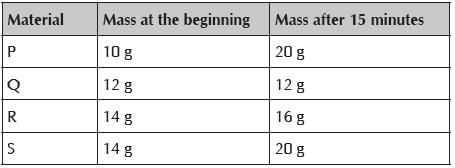Courses

# Olympiad Test: Matter - 1

## 10 Questions MCQ Test Science Olympiad for Class 5 | Olympiad Test: Matter - 1

Description
This mock test of Olympiad Test: Matter - 1 for Class 5 helps you for every Class 5 entrance exam. This contains 10 Multiple Choice Questions for Class 5 Olympiad Test: Matter - 1 (mcq) to study with solutions a complete question bank. The solved questions answers in this Olympiad Test: Matter - 1 quiz give you a good mix of easy questions and tough questions. Class 5 students definitely take this Olympiad Test: Matter - 1 exercise for a better result in the exam. You can find other Olympiad Test: Matter - 1 extra questions, long questions & short questions for Class 5 on EduRev as well by searching above.
QUESTION: 1

Solution:
QUESTION: 2

Solution:
QUESTION: 3

### Four thin sheets of material P, Q, R, and S of similar sizes were weighed individually. Then they were put into four beakers containing equal amounts of water. After 15 minutes, each sheet was weighed again. Their masses were recorded in the table below.Q. Which material absorbs the most amount of water?

Solution:
QUESTION: 4

Four thin sheets of material P, Q, R, and S of similar sizes were weighed individually. Then they were put into four beakers containing equal amounts of water. After 15 minutes, each sheet was weighed again. Their masses were recorded in the table below.Q. Which material will be most suitable for making a tent?

Solution:
QUESTION: 5

0 degree Celsius: Freezing point of water____________ : Boiling point of water

Solution:
QUESTION: 6

A substance changes from a solid to a liquid. Which point it has reached?

Solution:
QUESTION: 7

Which of the following is true for the molecules of gases?

Solution:
QUESTION: 8

When heat is supplied to molecules, their ___________ energy increases.

Solution:
QUESTION: 9

Pick the correct option.

Solution:
QUESTION: 10

Molecules are the components of:

Solution: